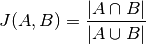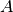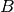Shortcuts

# Jaccard Index¶

## Module Interface¶

class torchmetrics.JaccardIndex(num_classes, ignore_index=None, absent_score=0.0, threshold=0.5, multilabel=False, reduction='elementwise_mean', compute_on_step=None, **kwargs)[source]

Computes Intersection over union, or Jaccard index:Where:andare both tensors of the same size, containing integer class values. They may be subject to conversion from input data (see description below). Note that it is different from box IoU.

Works with binary, multiclass and multi-label data. Accepts probabilities from a model output or integer class values in prediction. Works with multi-dimensional preds and target.

Forward accepts

• preds (float or long tensor): (N, ...) or (N, C, ...) where C is the number of classes

• target (long tensor): (N, ...)

If preds and target are the same shape and preds is a float tensor, we use the self.threshold argument to convert into integer labels. This is the case for binary and multi-label probabilities.

If preds has an extra dimension as in the case of multi-class scores we perform an argmax on dim=1.

Parameters
• num_classes (int) – Number of classes in the dataset.

• ignore_index (Optional[int]) – optional int specifying a target class to ignore. If given, this class index does not contribute to the returned score, regardless of reduction method. Has no effect if given an int that is not in the range [0, num_classes-1]. By default, no index is ignored, and all classes are used.

• absent_score (float) – score to use for an individual class, if no instances of the class index were present in preds AND no instances of the class index were present in target. For example, if we have 3 classes, [0, 0] for preds, and [0, 2] for target, then class 1 would be assigned the absent_score.

• threshold (float) – Threshold value for binary or multi-label probabilities.

• multilabel (bool) – determines if data is multilabel or not.

• reduction (Literal[‘elementwise_mean’, ‘sum’, ‘none’, None]) –

a method to reduce metric score over labels:

• 'elementwise_mean': takes the mean (default)

• 'sum': takes the sum

• 'none': no reduction will be applied

• compute_on_step (Optional[bool]) –

Forward only calls update() and returns None if this is set to False.

Deprecated since version v0.8: Argument has no use anymore and will be removed v0.9.

• kwargs (Dict[str, Any]) – Additional keyword arguments, see Advanced metric settings for more info.

Example

>>> from torchmetrics import JaccardIndex
>>> target = torch.randint(0, 2, (10, 25, 25))
>>> pred = torch.tensor(target)
>>> pred[2:5, 7:13, 9:15] = 1 - pred[2:5, 7:13, 9:15]
>>> jaccard = JaccardIndex(num_classes=2)
>>> jaccard(pred, target)
tensor(0.9660)


Initializes internal Module state, shared by both nn.Module and ScriptModule.

compute()[source]

Computes intersection over union (IoU)

Return type

Tensor

## Functional Interface¶

torchmetrics.functional.jaccard_index(preds, target, num_classes, ignore_index=None, absent_score=0.0, threshold=0.5, reduction='elementwise_mean')[source]

Computes Jaccard indexWhere:andare both tensors of the same size, containing integer class values. They may be subject to conversion from input data (see description below).

Note that it is different from box IoU.

If preds and target are the same shape and preds is a float tensor, we use the self.threshold argument to convert into integer labels. This is the case for binary and multi-label probabilities.

If pred has an extra dimension as in the case of multi-class scores we perform an argmax on dim=1.

Parameters
• preds (Tensor) – tensor containing predictions from model (probabilities, or labels) with shape [N, d1, d2, ...]

• target (Tensor) – tensor containing ground truth labels with shape [N, d1, d2, ...]

• num_classes (int) – Specify the number of classes

• ignore_index (Optional[int]) – optional int specifying a target class to ignore. If given, this class index does not contribute to the returned score, regardless of reduction method. Has no effect if given an int that is not in the range [0, num_classes-1], where num_classes is either given or derived from pred and target. By default, no index is ignored, and all classes are used.

• absent_score (float) – score to use for an individual class, if no instances of the class index were present in preds AND no instances of the class index were present in target. For example, if we have 3 classes, [0, 0] for preds, and [0, 2] for target, then class 1 would be assigned the absent_score.

• threshold (float) – Threshold value for binary or multi-label probabilities.

• reduction (Literal[‘elementwise_mean’, ‘sum’, ‘none’, None]) –

a method to reduce metric score over labels.

• 'elementwise_mean': takes the mean (default)

• 'sum': takes the sum

• 'none' or None: no reduction will be applied

Returns

Tensor containing single value if reduction is ‘elementwise_mean’, or number of classes if reduction is ‘none’

Return type

IoU score

Example

>>> from torchmetrics.functional import jaccard_index
>>> target = torch.randint(0, 2, (10, 25, 25))
>>> pred = torch.tensor(target)
>>> pred[2:5, 7:13, 9:15] = 1 - pred[2:5, 7:13, 9:15]
>>> jaccard_index(pred, target, num_classes=2)
tensor(0.9660)


© Copyright Copyright (c) 2020-2022, PyTorchLightning et al... Revision d27f1710.

Built with Sphinx using a theme provided by Read the Docs.
Versions
latest
stable
v0.8.1
v0.8.0
v0.7.3
v0.7.2
v0.7.1
v0.7.0
v0.6.2
v0.6.1
v0.6.0
v0.5.1
v0.5.0
v0.4.1
v0.4.0
v0.3.2
v0.3.1
v0.3.0
v0.2.0
v0.1.0